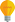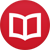# Can mathematical theorems be proved with 100% certainty?

by Jakub MarianTip: See my list of theMost Common Mistakes in English. It will teach you how to avoid mis­takes with com­mas, pre­pos­i­tions, ir­reg­u­lar verbs, and much more.

Many a mathematician considers mathematics to be the only truly exact science and would like to believe that the answer to the question in the title of this article is “yes”. They believe that mathematics is something independent of our minds, i.e. there doesn’t have to be any human being for it to work; it is just there.

This approach usually stems from a belief that our mathematics is somehow fundamental. (Most) mathematicians and physicists imagine the “computer of the universe” (i.e. the process that makes the laws of nature work) as already operating with our mathematical notions, especially natural numbers and logic.

However, there are good reasons to believe that this is in fact not the case; see my article about existence of natural numbers. It may happen that the “computer of the universe” uses a different kind “mathematics” which cannot be formulated using our language and mathematical notions, because our cognitive processes are fundamentally unable to construct and comprehend such a language.

But let’s suppose for the sake of argument that there really is something like “mathematics”. It may be just an incomplete way to describe reality, but let’s assume that it is already part of reality in some objective sense (as a sort of approximation).

Suppose you have a set of axioms, a theorem about some notions defined using the axioms, and a mathematician who has written a proof of that theorem. Can you be 100% sure that the theorem is true?

## Computers are able to check the proof, right?

Until there were computers, theorems were considered proven if mathematicians agreed that the proof they were presented with was correct (and, in fact, this is still usually the case). This doesn’t sound very objective, and indeed, there were cases when most mainstream mathematicians considered a theorem proven, although the proof turned out to be wrong later.

Mathematicians of that time knew they couldn’t know for sure that a theorem was correct. They just tried to do their best to find out what really was true and what wasn’t, always leaving room for possible errors. But after the invention of computers, methods for completely automatized proof checking became available.

What you have is a piece of software able to work with elementary logical and mathematical operations which is able to decide whether an implication is true or not according to the rules of logic. All you have to do is to translate the theorem in question and its proof to this rudimentary language, run the program, and let it decide.

At first sight, this approach sounds life-saving because it eliminates the need for a subjective decision. But then there is a question: how do we know that the decision the program makes is correct? Well, we know it, because we have proved it. Someone analysed the source code of the program and has proved that it did what it was supposed to do. How can we be sure that this proof was correct? We can’t.

There’s exactly the same level of uncertainty about the correctness of the program as there is about correctness of the theorem itself. It’s true that it reduces the “probability” that the proof is wrong, because the program is in a way another subject that checks it, but this still doesn’t give us the sought-for 100% certainty.

If we somehow knew that the program was 100% correct (or we were for some reason completely convinced that it was), could we then be sure that, if it says that a proof is correct, it really is?

The answer is yet again no. The computer that runs the program is part of the physical world and as such it is subject to outer interference. It is possible that each time we run the program, a highly energetic particle comes from outer space, hits the processor of the computer, and changes the calculation in such a way that the program says that the proof is correct, although it is not.

The conclusion is that while mathematics (resp. logic) undoubtedly is more exact than any other science, it is not 100% exact. We cannot be 100% sure that a mathematical theorem holds; we just have good reasons to believe it. As any other science, mathematics is based on belief that its results are correct. Only the reasons for this belief are much more convincing than in other sciences.

By the way, I have written several educational ebooks. If you get a copy, you can learn new things and support this website at the same time—why don’t you check them out?